Next Article in Journal
A Built Heritage Information System Based on Point Cloud Data: HIS-PC
Open AccessArticle

# A Machine Learning-Based Approach for Spatial Estimation Using the Spatial Features of Coordinate Information

Korea Institute of Geoscience and Mineral Resources (KIGAM), Daejeon 34132, Korea
*
Author to whom correspondence should be addressed.
ISPRS Int. J. Geo-Inf. 2020, 9(10), 587; https://doi.org/10.3390/ijgi9100587
Received: 31 August 2020 / Revised: 25 September 2020 / Accepted: 4 October 2020 / Published: 6 October 2020

## Abstract

With the development of machine learning technology, research cases for spatial estimation through machine learning approach (MLA) in addition to the traditional geostatistical techniques are increasing. MLA has the advantage that spatial estimation is possible without stationary hypotheses of data, but it is possible for the prediction results to ignore spatial autocorrelation. In recent studies, it was considered by using a distance matrix instead of raw coordinates. Although, the performance of spatial estimation could be improved through this approach, the computational complexity of MLA increased rapidly as the number of sample points increased. In this study, we developed a method to reduce the computational complexity of MLA while considering spatial autocorrelation. Principal component analysis is applied to it for extracting spatial features and reducing dimension of inputs. To verify the proposed approach, indicator Kriging was used as a benchmark model, and each performance of MLA was compared when using raw coordinates, distance vector, and spatial features extracted from distance vector as inputs. The proposed approach improved the performance compared to previous MLA and showed similar performance compared with Kriging. We confirmed that extracted features have characteristics of rigid classification in spatial estimation; on this basis, we conclude that the model could improve performance.

## 1. Introduction

The Kriging technique  is a method of estimating attribute data for unknown locations using known data. It has been established as a mathematical model by many scientists and has emerged as a representative methodology for geostatistics [2,3,4,5].
Recently, spatial estimation methodologies using a machine-learning approach (MLA) have been actively proposed. In particular, the random forest algorithm , which makes it relatively simple to control hyper-parameters and is easy to access through the development of packages [7,8], has been used as a representative technique for spatial estimation [9,10,11]. Initially, spatial coordinates were used in MLA to reflect the location information necessary for spatial estimation. However, when they were used only in the form of coordinates to learn location information, the results ignored spatial pattern appearing at the sample point. For this reason, the predicted attribute values in the model had a tendency to be overestimated or underestimated.
To overcome these problems with using the coordinate form, Hengl et al.  used the distances between all observation points, instead of the coordinate form, as the input of the algorithm so that the model could reflect the spatial relationship. The performances of spatial estimation were improved, and more stable estimations were obtained than before, when spatial correlation was considered. Nevertheless, if the sample data are small, these approaches have the disadvantage that performance may be lower than that of using Kriging owing to a lack of training data. Conversely, even if there are thousands or more items of sample data, there the input variable obtained by calculating the distance increases rapidly such that computing costs soar. This can lead to the curse of dimensionality—a common problem in machine learning. Moreover, the spatial dependence of data should be considered when applying the MLA for spatial estimation properly.
In general, training and test datasets are divided through a random splitting technique to verify the training performance in machine learning. However, as spatial data mostly have a local bias, if the data set is separated without considering spatial dependency, the estimation model may be suitable only for a specific local region of the entire dataset or significant error may occur in the verification of prediction performances [13,14]. Therefore, it is essential that partitioned spatial data should be composed evenly over the entire region [15,16].
In this study, we developed an MLA framework that considers the characteristics of spatial data based on what has been studied so far. In this framework, to consider issues that can occur when training spatial data using distance variables, the process of extracting spatial features from distance variables is included. In addition, we selected the random forest algorithm, which previous studies have found to have a robust performance in spatial estimation among various ML techniques [9,10,11,17], as a representative algorithm to focus on improving performance through training of spatial features extracted from input coordinate data. We expect that the extracted spatial features improve the performance of MLA for spatial estimation because they have characteristics of spatial correlation represented by distances. To verify the expected effect of the proposed approach, the Meuse dataset, which is publicly available, was used. A borehole dataset from Seoul, South Korea, was also used to verify the field applicability of the framework. In addition, instead of using other covariate variables in the spatial model, only coordinates were used as input variables in order to focus on comparing the effects of their transformation on the performance of estimation.

## 2. Theory and Background

#### 2.1. Indicator Kriging (IK)

Among the geostatistical techniques for spatial estimation, indicator Kriging (IK)  is a non-parametric approach that can be applied when the sample dataset is skewed or when it does not have a normal distribution. In addition, the IK does not directly predict the unknown target value but yields a set of K probability estimates [18,19] given by:
where n represents the number of available observations for displaying some degree of spatial correlation at location x, $z k$ is the kth threshold discretizing the range of variation of the attribute value z, and FIK is the conditional cumulative distribution function for IK.
To apply IK to spatial estimation, target attribute values should be converted to indicators according to a certain number of threshold values. The indicators are coded as binary functions and are transformed into continuous or categorical indicators according to the data type. Equation (2) shows the indicator transformation for continuous data:
where $x a$ is the observation location.
As with the ordinary Kriging process, variogram modelling should be conducted using the transformed indicators. The calculated indicator values are converted to the spatial attribute values using the conditional cumulative probability distribution based on each threshold value. Values between the threshold values are mainly calculated using linear interpolation; another approximation method can be used when the differences between threshold values are large [4,5,20].

#### 2.2. Random Forest (RF)

The random forest (RF) [6,21] approach, which solves a problem by learning multiple decision trees, is a representative ensemble technique and data-based statistical method. The decision tree technique is an unreliable solution because the performance of prediction varies greatly depending on the training data; furthermore, it is also prone to overfitting its training data. To overcome these issues, bagging and boosting methods, which are ensemble techniques that consider multiple decision trees to train data, have been developed and studied. Bagging is a method of aggregating basic trees; it is trained for each dataset and is created through the bootstrap process to develop a dataset of the same size while allowing redundancy in the sample dataset. Therefore, it can be said to be a parallel ensemble model that learns each model independently, and has the characteristics of reducing the variance and avoiding overfitting of the predicted model. RF uses almost the same framework as bagging, but the one difference is that it randomly selects and uses the feature at the split branch of the node [6,21,22]. The values predicted through bagging can be expressed as the average values of the predicted values of individual trees as:
$θ ^ B ( x ) = 1 B ∑ b = 1 B t b * ( x )$
where b is the individual bootstrap sample, B is the total number of b, $t b *$ is the individual decision tree for sample b, and:
where $c b k *$ is the kth training sample with pairs of values for the response (y) and predictors (x): $c b k * = ( x k , y k )$.
As the RF can train data without complicated adjustment of the hyper-parameters and can be applied to multiple class problems without restriction, it is used for various regression and classification problems in geoscience fields.

#### 2.3. Principal Component Analysis (PCA)

PCA [23,24] is a multivariate analysis method that uses the relationships between variance and covariance of quantitative variables to find the principal components (PCs) and to roughly describe the overall variation of the original data. As PCA finds a basis orthogonal to each other, while preserving the variance as much as possible, it is possible to transform a high-dimensional space into a low-dimensional space without linear correlation. Each PC is calculated to minimize the loss of information of original data . When PCA is applied to the data, a weight vector for kth PC is obtained as:
where w is the unit weight vector and $X ^ k$ is the dataset subtracting k-1th PC from the original dataset $X$; $X ^ k$ is given by:
$X ^ k = X − ∑ i = 1 k − 1 w i w i T X$
where $X ^ 1$ is the same as the original dataset $X$.
As PCA can be applied for pre-processing and visualization of high-dimensional data sets, it is used as a representative dimensional reduction method in various research fields. In regression problems where explanatory variables with strong correlation exist, PCA can reduce the number of variables and improve the regression performance . In addition, it is used for the extraction of the main separation variables in cluster analysis [27,28] or in the processing of data with high noise [29,30].

## 3. Methodology

In general, estimators based on second-order moments, such as Kriging, need assumptions for second-order and intrinsic stationarity, which should be predefined in a two points statistics model. In the process of the variogram modelling that reflects these stationary hypotheses, specialized knowledge that can involve the subjectivity of experts is necessary to set parameters of a variogram. In contrast, the MLA as a proposed method in this study for spatial estimation does not require and assumption for stationary hypotheses of data and variogram modelling. However, if the spatial data is trained without additional pre-processing and consideration spatial bias, it is possible for the prediction results to ignore spatial autocorrelation. Figure 1 shows the process of Kriging and that of the proposed methodology for spatial estimation. When applying Kriging to spatial problems, it is essential to find the normality of target attribute data. If the distribution of it is not normal distribution and skewed, it should be transformed because the Kriging estimator is sensitive to large data values. There are two typical methods for applying Kriging to spatial data with such skewness. The first is to apply ordinary Kriging after data transformation. In this case, Box–Cox transformation including logarithmic transformation is typically used to transform the data. However, problematically, Kriging results are biased when the data is back-transformed through application of the inverse of the original transformation to the Kriging estimates of the transformed data. The second is to use IK without considering back-transformation. However, to apply IK, the data must be transformed into separate indicators based on specific thresholds, and variograms for each indicator should be modeled separately. After considering these points, variogram modelling using the converted data was conducted, and spatial estimation was performed by applying the theoretical variogram model to the Kriging calculation. During this process, the results of data transformation and variogram modelling are different, according to expert knowledge, which affects performances of spatial estimation. In contrast, Figure 1b shows the process of spatial estimation through the MLA, which is divided into four main steps: (i) data preparation and processing; (ii) data partitioning; (iii) selecting the machine-learning algorithm and hyper-parameter optimization, and (iv) training and estimating spatial data.

#### 3.1. Data Preparation & Processing

Spatial estimation based on the MLA is also a coordinate-based data-inference process similar to Kriging. The target attribute value (e.g., the concentration of pollutants, deposits of minerals and the thickness of layers) is set as the output of the MLA. For input, location information (e.g., coordinates, altitude) are used. Although other covariates affecting estimation of the target values can be included, we used coordinates as inputs to compare the performances of spatial prediction according for different methodologies under the same data conditions. Conventionally, when using the MLA for spatial estimation, coordinates are used without deformation. However, recently, to mimic the spatial correlation used in Kriging, a distance vector that calculates distances between the point to be predicted and all sample points have been used [12,17]. Although there are various types of distance calculation algorithm, we used Euclidean distance for considering spatial correlation. Conversely, when transforming raw coordinates into a distance vector, the number of input variables increases by the number of sample points. For example, if there are 10 sample points, a 10 × 10 distance matrix is calculated, and each sample point has distance vector which includes 10 distance variables. However, if there are 1000 sample points, the distance vector to be used as an input will have 1000 variables. In this case, the computational complexity of the MLA increases exponentially, which can increase computation time and reduce performance. Therefore, the dimension of the input variable was reduced by applying PCA to reduce the computational complexity of the MLA.

#### 3.2. Data Partitioning

For training and evaluating the performances of MLA, sample data should be divided into a training dataset and a test dataset. To address issues with a large amount of sample data, it is common to divide training and test datasets according to a certain ratio, the so-called hold-out validation method. However, when there are a small number of sample data points, training performance can be significantly reduced depending on the data separation ratio; hence, the k-fold validation method is generally used. As the method consists of k data partitions and performs training and validation k number of times, this method has the advantage of evaluating the prediction performance of the algorithm for the entire data set even with a small amount of data. When applying this method to spatial data, the individual fold partition should be composed in such a way so as to consider the entire area that needs to be estimated .

#### 3.3. Machine Learning Algorithm and Hyper-Parameter Optimization

Various machine-learning algorithms have been used to solve regression and classification problems of spatial data. For example, RF algorithms have many variants that can be used for spatial regression problems. We used quantile RFs  that can calculate the variance of prediction error as well as the target attribute values of a spatial region. In the MLA, as the training performance of the algorithm varies depending on the hyper-parameters, parameter optimization is necessary. In RF, the number of trees, the size of minimum leaf and the number of features for the split node are the typical hyper-parameters. In the case of the number of trees, the RF becomes more robust to overfitting when it increases, but the increase in performance becomes very small above a certain number. Therefore, it is recommended to set it to a large number within the limit that can be calculated in the researcher’s computer environment . Meanwhile, hyper-parameter optimization was performed through the grid search method for setting the size of minimum leaf and the number of features for the split node in this study.

#### 3.4. Training and Estimation of Spatial Data

When the processing of the spatial data and the overall setting of the machine-learning algorithm were completed, training and estimation of the data were performed using the spatial MLA. In this study, the k-fold validation method was performed on a sample dataset to evaluate the prediction performance, and the spatial estimation of unknown locations was then conducted using the trained model.

## 4. Experiment

This section describes the experiment conducted to compare the results of spatial estimation using the Kriging and the MLA. The distributions of target attribute values in datasets to be estimated, which is described in more detail in Section 4.1, have high positive skewness and even have an attribute value of zero. In consideration of the characteristics of these data, IK, which does not require back-transformation, was used for conventional spatial estimation. For modelling the indicator variogram and applying IK, an auto-IK software package  was used. For the MLA, the ‘TreeBagger’ library that is included in the statistics and machine learning toolbox of MATLAB 2018 was used. In addition, the experiment was conducted on an Intel Core i7-9700K 3.60 GHz processor and 64 GB RAM specification.

#### 4.1. Dataset

The Meuse dataset , which is publicly available, was used to evaluate the performance of spatial prediction, and the Seoul borehole dataset was used to verify the field applicability of the proposed method. The borehole dataset contains information related to the thickness of the deposited soil, in Seoul, South Korea (37°41′33″–37°71′51″ N, 126°73′41″–127°26′93″ E), surveyed for the development of underground infrastructure. It was acquired from the open geotechnical survey information provided by the Korean Geotechnical Information DB system. It consisted of borehole code, location information (coordinate and altitude), stratum code, stratum start depth, stratum end depth, stratum thickness and stratum name. The stratum was classified according to the standard ground classification of the Seoul. In this study, information on the thickness data of deposit soil in 400 boreholes was used as a dataset for spatial estimation. Figure 2 shows a Meuse dataset with 155 sample points. Figure 2a shows the distribution of zinc concentrations and Figure 2b shows the histogram and basic statistics of the zinc concentrations. Figure 3a shows the distribution of deposit soil thickness included in 400 borehole data. Figure 3b shows the histogram and basic statistics of the thicknesses. When analyzing the histograms and statistics, both datasets are similar. The distributions of the attribute values have high positive skewness, which means that they do not have a normal distribution. In addition, most sampled points have low attribute values, and a few points have extremely high values.

#### 4.2. Experimental Setup

To compare the performances of spatial estimation, the algorithms and characteristics of each method are presented in Table 1. As the datasets used for spatial estimation are not normally distributed, the spatial attribute values should be converted to a Box–Cox transformation or indicators to apply the Kriging. In this study, IK was selected as a comparative reference method considering the data distribution and the characteristics, including the zero value in the borehole dataset. The RF was applied for the spatial estimation based on the MLA. In addition, the MLA was divided into three methods depending on the type of transformed input and by applying the PCA.
In general, hyper-parameters that require optimization of the RF are the size of the minimum leaf, the number of features for the split node, and the number of trees. A detailed description of the fine-tuning of hyper-parameters is given in Kuhn and Johnson . In this study, the number of trees was set to 500 so that the RF algorithm can be robust against overfitting through trial and error. In the case of the size of minimum leaf and the number of features for the split node, optimization was performed through the grid search method. The minimum leaf size is set to five as a general default setting for the RF for regression. In this study, the intervals from one to five are set as intervals for grid search of the variable. Meanwhile, the number of features for the split node is set to one third of the total number of input variables for the default setting. We used spatial features extracted from the distance vector as an input variable for MLA spatial estimation. Therefore, in the process of reducing the dimension of the input variable by applying PCA, the optimal number of extracted components varies depending on the dataset. Due to this, the grid search interval to optimize the number of features for the split node was set differently for each case. For example, in the case of the number of dimensions reduced to PCA is fifteen in the Meuse Dataset, the interval of the number of feature for the split node is set to 1, 3, 6, 9, 12 and 15, and the interval of the minimum leaf size is set from one to five as a grid search interval. Therefore, the optimal hyper-parameters were set by comparing the results for a total of thirty cases. In addition, the results of a hundred iterations per grid search case were compared to ensure reliability for performance.

#### 4.3. Cross-Validation Method

In this study, k-fold cross-validation was used because the number of sample data in both datasets was not large enough to perform hold-out validation. The datasets were divided into five folds, and the data of each fold were not biased in a specific area to avoid extrapolation issue in spatial prediction as much as possible (Figure 4).

#### 4.4. Model Performance Criteria

To compare the performances of the IK and the MLA, R-squared (Equation (7)) and root mean squared error (RMSE) (Equation (8)) were used for performance criteria, along with basic statistics such as average, minimum, and maximum values. Each method was compared through the performances based on predicted attribute values from all sample points generated by five-fold cross-validation. R-squared is given by:
$R 2 = 1 − S S r e s i d u a l s S S t o t a l$
where $S S r e s i d u a l s$ is the sum of squared errors at cross-validation points and $S S t o t a l$ is the total sum of squares (i.e., the sum of squared differences between the sample point and their mean).
where $y ^ ( x a )$ is the predicted value of $y$ at cross-validation point $x a$ and $n$ is the total number of cross-validation points.

## 5. Results and Discussion

#### 5.1. Variogram Modelling for IK

Variogram modelling was performed for IK, a benchmark model that provides a standard for comparing the performance of the methodologies. An indicator transformation was conducted on both datasets to perform the variogram modelling. Nine percentiles, separated according to nine thresholds, were applied for the indicator transform. Figure 5 and Figure 6 show the calculated experimental variograms and the modeled theoretical variogram for the Meuse and Seoul borehole datasets, respectively. Exponential, spherical, and Gaussian models were used to compute the theoretical variograms. The parameters of each theoretical variogram are presented in Table 2. In the case of indicator variogram modelling for the Seoul borehole dataset, the same variogram from threshold one to threshold four is calculated, as shown in Figure 4, because forty percent of the target attribute value is zero.

#### 5.2. Optimization of the Number of PCs

Before comparing the performance of each method, the process of selecting the optimal number of PCs was conducted by evaluating the prediction performance according to the number of PCs. Each performance was evaluated through five-fold cross-validation. R-squared and RMSE were used as performance criteria. Figure 7 shows the results of evaluating the R-squared and RMSE according to the number of PCs in the Meuse and Seoul datasets. As the results predicted from each tree are random in the RF, the prediction can be different even though the algorithm consists of five hundred trees. Therefore, a hundred RFs were performed according to the number of PCs, and each performance was shown in a box-plot. As a result, the best performance was obtained when the number of PCs was fifteen and twelve for the Meuse and Seoul borehole datasets, respectively. Finally, the performance of the proposed method, using the optimal number of PCs, was compared with the performance of other methods.

#### 5.3. Validation of Prediction Performances

The prediction performances of each method are specified in Table 3. Figure 8 shows a box-plot comparing the R-squared values of each method. For the RF spatial predictions, the performances are shown as a box-plot because different performances were calculated according to one hundred iterations. On the contrary, for the IK, a line in the box-plot is shown because the same result is obtained regardless of the number of iterations. Overall, when comparing the spatial prediction results of each method for both datasets, the range of target values (i.e., the difference between the maximum and minimum values) predicted by the IK was narrower than the range of true data, and the standard deviation was low. The reason for this is that IK applies ordinary Kriging for each indicator; therefore, the target values of the regions within each indicator are predicted to approximate the average of the individual thresholds.
Due to this local smoothing effect, the range of predicted values tends to appear narrow. On the contrary, the range of target attribute values and standard deviation predicted by applying RF were relatively higher than IK. In addition, the RF spatial estimations were superior to IK in predicting the local high values. The reason for this is that the RF can reflect stochastic uncertainty by predicting target values differently for each tree. The accuracy of spatial prediction, evaluated by R-squared, showed IK was relatively higher compared to RF. However, RF-PCA had the same or better accuracy than IK. As shown in Figure 8 and Table 3, the spatial prediction accuracy of RF-PCA was close to that of IK, 0.46, for the Seoul dataset. In addition, RF-PCA had a higher accuracy than IK with a spatial prediction accuracy of 0.62 for the Meuse dataset. The reason the accuracy of the spatial prediction of RF-PCA was higher than other RF methods is that the regression performance of RF was improved by using a certain number of PCs that had characteristics to explain the spatial relationship between observations. The effect of applying PCA to RF is described in detail in a later discussion section.

#### 5.4. Results of Mapping on Spatial Grids

To compare the performance of estimation for spatial uncertainty, the predicted attribute values were mapped to grids, with a certain distance, for both datasets. The spatial prediction was performed on 3103 grids spaced 40 m apart for the Meuse dataset and on 58,074 grids spaced 100 m apart for the Seoul dataset. Figure 9 and Figure 10 show the spatial prediction results and the standard deviations of the prediction errors for the Meuse and Seoul datasets, respectively. Overall, correlations between maps applying IK and RF methods were high (each correlation coefficient was higher than 0.9).
The attribute values of RF methods were predicted high near the local outliers, and the standard deviation of the prediction error was extremely high for both datasets, as shown in Figure 9 and Figure 10. The influence of local outliers was largely reflected in the spatial prediction of the RF methods. However, among RF methods, it is difficult to say that RF-Coord is an appropriate spatial estimation technique because it had not only relatively low prediction performance in validation but also had blocky artifacts. On the contrary, RF-Dist estimated spatial patterns smoothly, similar to IK. This can be inferred from the results of bagging, which averages the predicted results of individual trees while taking into account all distances of each observation point as predictor variables . RF-PCA has a weaker smoothing effect in spatial pattern than RF-Dist but is a locally separated trend. As shown in Figure 9e, the local trend appearing in the RF-PCA result is confirmed by the diagonal artifact crossing over the entire map for the Meuse dataset.

#### 5.5. Effects Using Extracted PCs for Spatial Prediction

The RF-PCA showed higher prediction performances in validation compared to other methods. However, it was found that artifacts might occur depending on the distribution trend of the target attribute values, as shown in the Meuse dataset (Figure 9e). The reason for these artifacts is that each PC extracted by applying PCA to spatial data has a rigid spatial classification characteristic. To support this, it was confirmed that the diagonal direction artifact disappeared when spatial attribute values were estimated, excluding the third PC in the Meuse dataset, as shown in Figure 11.
Figure 11a is the RF result of setting the fifteen PCs, including the third PC as input, and Figure 11b is the RF result of setting the input after excluding the third PC in the fifteen PCs. With mapping results, R-squared was 0.61 when the third PC was included, but if it was excluded, the performance was lowered to 0.54. In conclusion, the third PC has a classification characteristic about the distribution of zinc concentrations, which improves the prediction performance of the RF. However, its rigorous classification characteristic creates artifacts in spatial mapping. This can also be seen as a problem that can occur when learning variables with strong discrimination for predicting values are in a tree-based approach. Therefore, it is necessary to figure out whether visible artifacts are generated when an MLA that is not tree-based is applied in future works.
On the contrary, as the number of PCs used in the RF excessively increases, the accuracy of spatial prediction decreases, and therefore the target values were underestimated. Figure 12 shows the prediction results in a scatter plot after five-fold cross-validation for both datasets according to the number of PCs. As the number of PCs increased, the slope of the trend line with zero intercept decreased. Through this, it was assumed that the RF was underestimated. In addition, the underestimated prediction trend was confirmed by the gradual decrease of the average and maximum values of the predicted results, as described in Table 4.
It is assumed that this effect occurs because the PCs extracted in a late order have the low explainable ability for the original data. PCs have characteristics only for regions with low attribute values that make up the majority of the data in both datasets. Accurately adding components for spatial estimation can increase the performances to classify and predict the target values of the dataset, such as fine-tuning effects. However, if PCs are added excessively, the variable that can explain low attribute values in the algorithm becomes dominant. This reduces the explainable ability for local outliers with high attribute values in the algorithm and causes the trained model to underestimate.

## 6. Conclusions

Recently, research cases for spatial estimation through application of MLA in addition to the traditional geostatistical techniques are increasing. For the purpose of improving the spatial estimation performance of MLA, this study focused on comparing the difference in performance according to the transformation type of coordinates, which can be considered as basic inputs in spatial estimation. We proposed a methodology that uses spatial features extracted from the distance vector. As a result, MLA showed prediction performances and the results of spatial mapping similar to those of Kriging. It is worth noting that the spatial estimation performance can be improved while solving the problem of increasing complexity for spatial estimation of MLA due to the use of the distance vector proposed in the previous study. A summary of the strengths of the proposed methodology are as follows:
• Spatial estimation through MLA does not require assumptions about stationarity and variogram modelling. Moreover, additional transformation and back transformation for target variables are not required.
• Spatial correlation of data could be considered by using the distance vector as an input in the MLA. By applying PCA to the distance vector, it was possible to reduce the complexity of the input variable. Due to this, the computational cost of MLA is reduced, and the spatial estimation performance could increase.
• These results were obtained using only the coordinates for spatial estimation, without the addition of other covariates. Therefore, the proposed methodology can be used as a method for improving the performance of estimation in problems where there is no information available other than location information of sample data when using MLA.
The proposed method has the above-mentioned advantages, but suffers from issues relating to spatial mapping, which need to be addressed through additional research, to improve the method into a more robust methodology. The issues are as follows:
• As a result of the application of the proposed methodology, the spatial estimation performance has improved, but artifacts have occurred according to the characteristics of the tree-based algorithm during the mapping process, which may vary depending on the spatial distribution of the target data. In future works, we should compare the results of applying the proposed method to MLA techniques other than RF, or study how to mitigate these effects in other ways.
• The computational cost of RF was reduced by applying PCA, but a direct comparison of computation cost was not conducted because a large dataset was not used. In future studies, we will apply the proposed method for a large point dataset and study the cost-effectiveness.
• The future studies can include the exploration of the application and effects of various techniques that can be used as a tool to extract spatial features other than PCA.

## Author Contributions

Overall conceptualization, data configuration and review, Dong-Woo Ryu; methodology, validation, performance evaluation and writing, Seongin Ahn; visualization and editing, Sangho Lee. All authors have read and agreed to the published version of the manuscript.

## Funding

This research was supported by the Basic Research Project of the Korea Institute of Geoscience and Mineral Resources (KIGAM) (GP2020-031;20-3117) funded by the Ministry of Science and ICT of Korea.

## Acknowledgments

We would like to thank the Ministry of Science and ICT of Korea for supporting this research.

## Conflicts of Interest

The authors declare no conflict of interest.

## References

1. Krige, D.G. A statistical approach to some basic mine valuation problems on the Witwatersrand. J. S. Afr. I. Min. Metal. 1951, 52, 119–139. [Google Scholar] [CrossRef]
2. Cressie, N. The origins of kriging. Math. Geosci. 1990, 22, 239–252. [Google Scholar] [CrossRef]
3. Isaaks, E.H.; Srivastava, R.M. An Introduction to Applied Geostatistics; Oxford University Press: New York, NY, USA, 1989; ISBN 978-0-1950-5013-4. [Google Scholar]
4. Goovaerts, P. Geostatistics for Natural Resources Evaluation; Oxford University Press: New York, NY, USA, 1997; ISBN 978-0-1951-1538-3. [Google Scholar]
5. Deutsch, C.V.; Journel, A.G. GSLIB: Geostatistical Software Library and User’s Guide, 2nd ed.; Oxford University Press: New York, NY, USA, 1998; ISBN 978-0-1951-0015-0. [Google Scholar]
6. Breiman, L. Random forests. Mach. Learn. 2001, 45, 5–32. [Google Scholar] [CrossRef]
7. Liaw, A.; Wiener, M. Classification and regression by random forest. R News 2002, 2, 18–22. [Google Scholar]
8. Wright, M.N.; Ziegler, A. Ranger: A fast implementation of random forests for high dimensional data in C++ and R. J. Stat. Softw. 2017, 77. [Google Scholar] [CrossRef]
9. Hengl, T.; Heuvelink, G.B.M.; Kempen, B.; Leenaars, J.G.B.; Walsh, M.G.; Shepherd, K.D.; Sila, A.; MacMillan, R.A.; de Jesus, J.M.; Tamene, L.; et al. Mapping soil properties of Africa at 250 m resolution: Random forests significantly improve current predictions. PLoS ONE 2015, 10, e0125814. [Google Scholar] [CrossRef]
10. Nussbaum, M.; Spiess, K.; Baltensweiler, A.; Grob, U.; Keller, A.; Greiner, L.; Schaepman, M.E.; Papritz, A. Evaluation of digital soil mapping approaches with large sets of environmental covariates. Soil 2018, 4, 1–22. [Google Scholar] [CrossRef]
11. Georganos, S.; Grippa, T.; Gadiaga, A.N.; Linard, C.; Lennert, M.; Vanhuysse, S.; Mboga, N.; Wolff, E.; Kalogirou, S. Geographical random forests: A spatial extension of the random forest algorithm to address spatial heterogeneity in remote sensing and population modelling. Geocarto Int. 2019. [Google Scholar] [CrossRef]
12. Hengl, T.; Nussbaum, M.; Wright, M.N.; Heuvelink, G.B.M.; Graler, B. Random forest as a generic framework for predictive modeling of spatial and spatio-temporal variables. PeerJ 2018, 6. [Google Scholar] [CrossRef]
13. Juel, A.; Groom, G.B.; Svenning, J.C.; Ejrnaes, R. Spatial application of random forest models for fine-scale coastal vegetation classification using object based analysis of aerial orthophoto and DEM data. Int. J. Appl. Earth Obs. 2015, 42, 106–114. [Google Scholar] [CrossRef]
14. Meyer, H.; Reudenbach, C.; Hengl, T.; Katurji, M.; Nauss, T. Improving performance of spatio-temporal machine learning models using forward feature selection and target-oriented validation. Environ. Model. Softw. 2018, 101, 1–9. [Google Scholar] [CrossRef]
15. Meyer, H.; Reudenbach, C.; Wöllauer, S.; Nauss, T. Importance of spatial predictor variable selection in machine learning applications—Moving from data reproduction to spatial prediction. Ecol. Model. 2019, 411. [Google Scholar] [CrossRef]
16. Valavi, R.; Elith, J.; Lahoz-Monfort, J.J.; Guillera-Arroita, G. BlockCV: An r package for generating spatially or environmentally separated folds for k-fold cross-validation of species distribution models. Methods Ecol. Evol. 2019, 10, 225–232. [Google Scholar] [CrossRef]
17. Behrens, T.; Schmidt, K.; Rossel, R.A.V.; Gries, P.; Scholten, T.; MacMillan, R.A. Spatial modelling with Euclidean distance fields and machine learning. Eur. J. Soil Sci. 2018, 69, 757–770. [Google Scholar] [CrossRef]
18. Journel, A.G. Nonparametric estimation of spatial distributions. Math. Geosci. 1983, 15, 445–468. [Google Scholar] [CrossRef]
19. Goovaerts, P. AUTO-IK: A 2D indicator kriging program for the automated non-parametric modeling of local uncertainty in earth sciences. Comput. Geosci. 2009, 35, 1255–1270. [Google Scholar] [CrossRef] [PubMed]
20. Remy, N.; Boucher, A.; Wu, J. Applied Geostatistics with SGeMS: A User’s Guide; Cambridge University Press: New York, NY, USA, 2009; ISBN 978-1-1074-0324-6. [Google Scholar]
21. Ho, T.K. The random subspace method for constructing decision forests. IEEE TPAMI 1998, 20, 832–844. [Google Scholar]
22. Hastie, T.; Tibshirani, R.; Friedman, J. The Elements of Statistical Learning: Data Mining, Inference, and Prediction, 2nd ed.; Springer: New York, NY, USA, 2013; ISBN 978-1-4614-6848-6. [Google Scholar]
23. Pearson, K. LIII. On lines and planes of closest fit to systems of points in space. London, Edinburgh Dublin Philos. Mag. J. Sci. 1901, 2, 559–572. [Google Scholar] [CrossRef]
24. Hotelling, H. Analysis of a complex of statistical variables into principal components. J. Educ. Psychol. 1933, 24, 417. [Google Scholar] [CrossRef]
25. Jolliffe, I.T. Principal Component Analysis, 2nd ed.; Springer: New York, NY, USA, 2002; ISBN 978-0-387-95442-4. [Google Scholar]
26. Wuttichaikitcharoen, P.; Babel, M. Principal component and multiple regression analyses for the estimation of suspended sediment yield in ungauged basins of Northern Thailand. Water 2014, 6, 2412–2435. [Google Scholar] [CrossRef]
27. Iwamori, H.; Yoshida, K.; Nakamura, H.; Kuwatani, T.; Hamada, M.; Haraguchi, S.; Ueki, K. Classification of geochemical data based on multivariate statistical analyses: Complementary roles of cluster, principal component, and independent component analyses. Geochem. Geophys. 2017, 18, 994–1012. [Google Scholar] [CrossRef]
28. Kang, B.; Jung, H.; Jeong, H.; Choe, J. Characterization of three-dimensional channel reservoirs using ensemble Kalman filter assisted by principal component analysis. Pet. Sci. 2019, 17, 182–195. [Google Scholar] [CrossRef]
29. Bailey, S. Principal component analysis with noisy and/or missing data. Publ. Astron. Soc. Pac. 2012, 124, 1015. [Google Scholar] [CrossRef]
30. Marinov, T.V.; Mianjy, P.; Arora, R. Streaming principal component analysis in noisy setting. In Proceedings of the 35th International Conference on Machine Learning, PMLR 2018, Stockholm, Sweden, 10–15 July 2018; Volume 80, pp. 3413–3422. [Google Scholar]
31. Meinshausen, N. Quantile regression forests. J. Mach. Learn. Res. 2006, 7, 983–999. [Google Scholar]
32. Probst, P.; Boulesteix, A.-L. To tune or not to tune the number of trees in random forest. J. Mach. Learn. Res. 2018, 18, 1–18. [Google Scholar]
33. Rikken, M.G.J. Soil Pollution with Heavy Metals: In Inquiry into Spatial Variation, Cost of Mapping and the Risk Evaluation of Copper, Cadmium, Lead and Zinc in the Floodplains of the Meuse West of Stein; The Netherlands: Field Study Report; University of Utrecht: Utrecht, The Netherlands, 1993. [Google Scholar]
34. Kuhn, M.; Johnson, K. Applied Predictive Modeling, 1st ed.; Springer: New York, NY, USA, 2013; ISBN 978-1-4614-6848-6. [Google Scholar]
Figure 1. Comparison of spatial estimation approaches. Schematic difference between (a) Kriging and (b) the machine-learning approach (MLA) for spatial estimation.
Figure 1. Comparison of spatial estimation approaches. Schematic difference between (a) Kriging and (b) the machine-learning approach (MLA) for spatial estimation.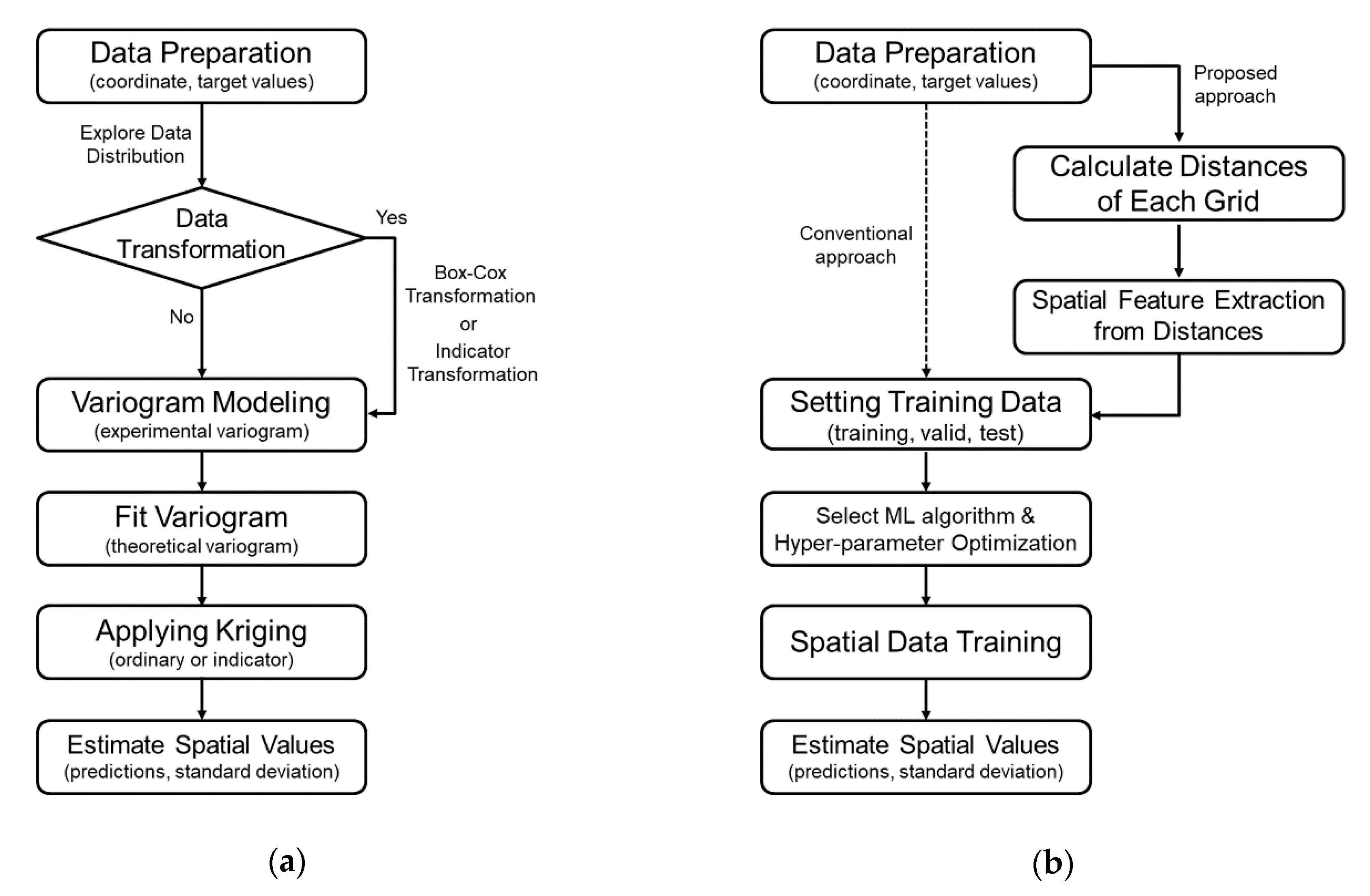Figure 2. Information about Meuse dataset: (a) a spatial distribution of zinc concentrations and (b) a histogram with basic statistics.
Figure 2. Information about Meuse dataset: (a) a spatial distribution of zinc concentrations and (b) a histogram with basic statistics.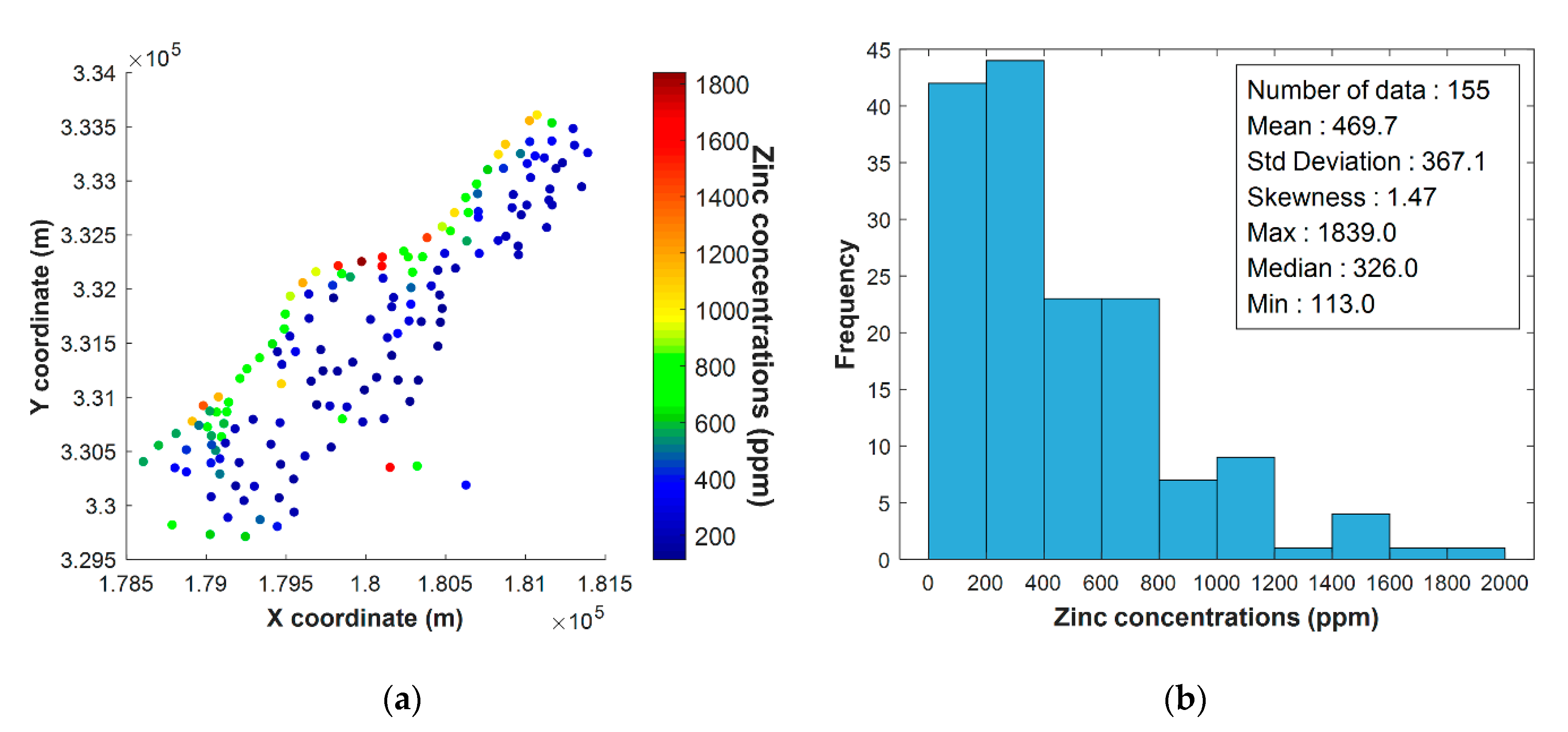Figure 3. Information about the Seoul borehole dataset: (a) a spatial distribution of the deposit soil thickness and (b) a histogram with basic statistics.
Figure 3. Information about the Seoul borehole dataset: (a) a spatial distribution of the deposit soil thickness and (b) a histogram with basic statistics.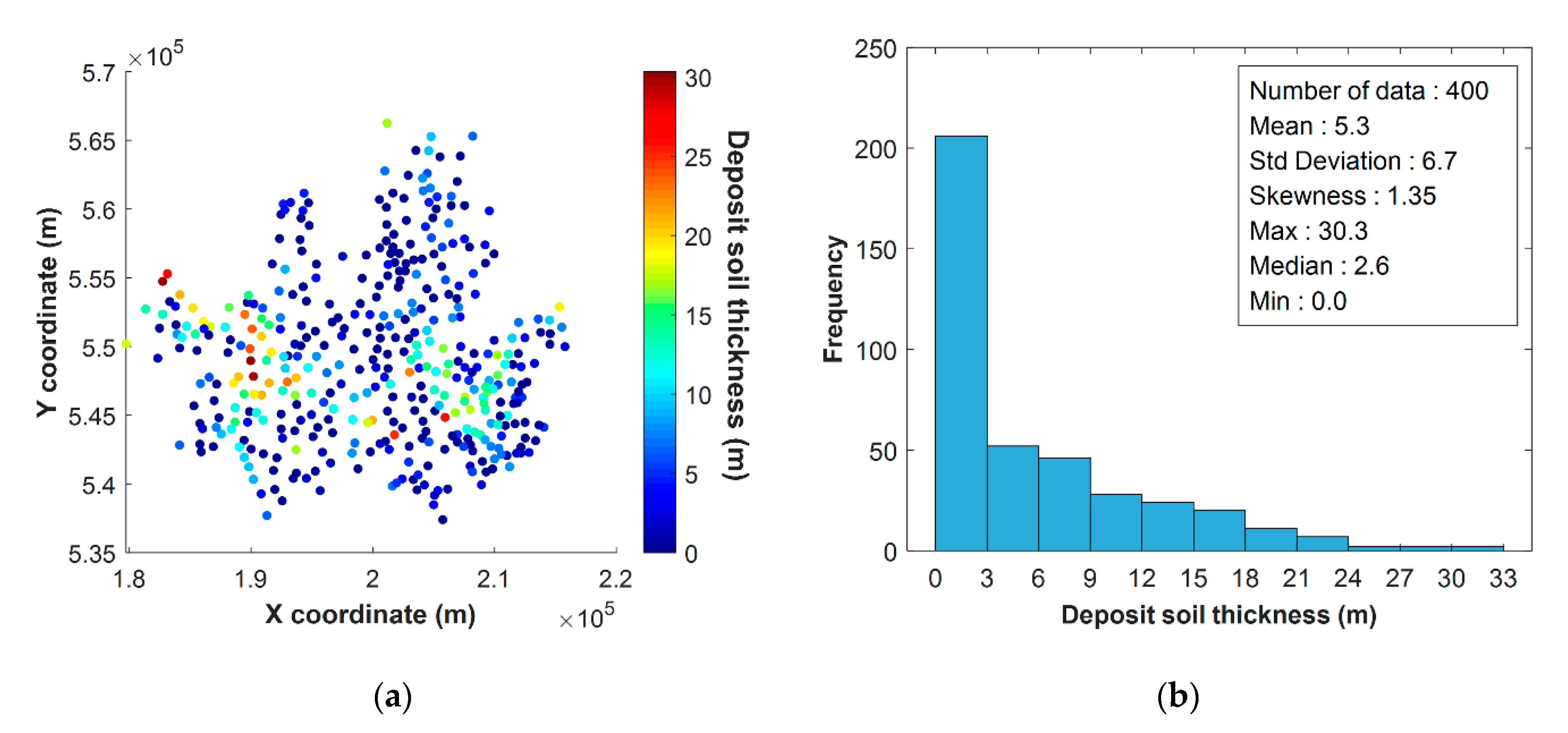Figure 4. Results of dividing sample data into five folds considering unbiased spatial distribution for (a) Meuse and (b) Seoul borehole datasets.
Figure 4. Results of dividing sample data into five folds considering unbiased spatial distribution for (a) Meuse and (b) Seoul borehole datasets.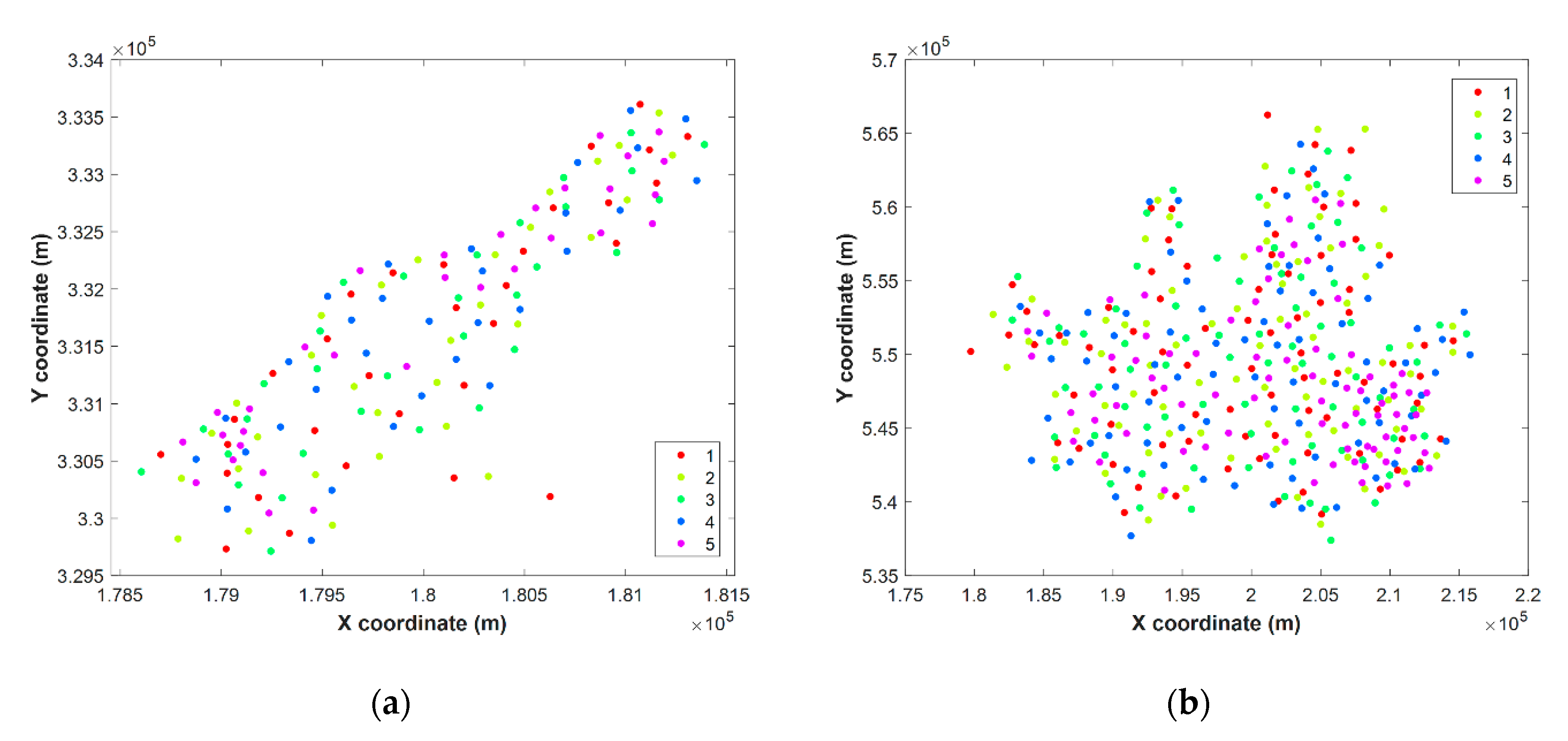Figure 5. Experimental (dot) and theoretical (line) variograms calculated for thresholds (a) one to (i) nine of the Meuse dataset.
Figure 5. Experimental (dot) and theoretical (line) variograms calculated for thresholds (a) one to (i) nine of the Meuse dataset.Figure 6. Experimental (dot) and theoretical (line) variograms calculated for thresholds (a) one to (i) nine of the Seoul borehole dataset.
Figure 6. Experimental (dot) and theoretical (line) variograms calculated for thresholds (a) one to (i) nine of the Seoul borehole dataset.Figure 7. Results of the performances of spatial estimation according to the number of principal components (PCs): (a) R-squared and (b) RMSE for the Meuse dataset; (c) R-squared and (d) RMSE for the Seoul dataset.
Figure 7. Results of the performances of spatial estimation according to the number of principal components (PCs): (a) R-squared and (b) RMSE for the Meuse dataset; (c) R-squared and (d) RMSE for the Seoul dataset.Figure 8. Prediction performance results: (a) Meuse dataset and (b) Seoul dataset.
Figure 8. Prediction performance results: (a) Meuse dataset and (b) Seoul dataset.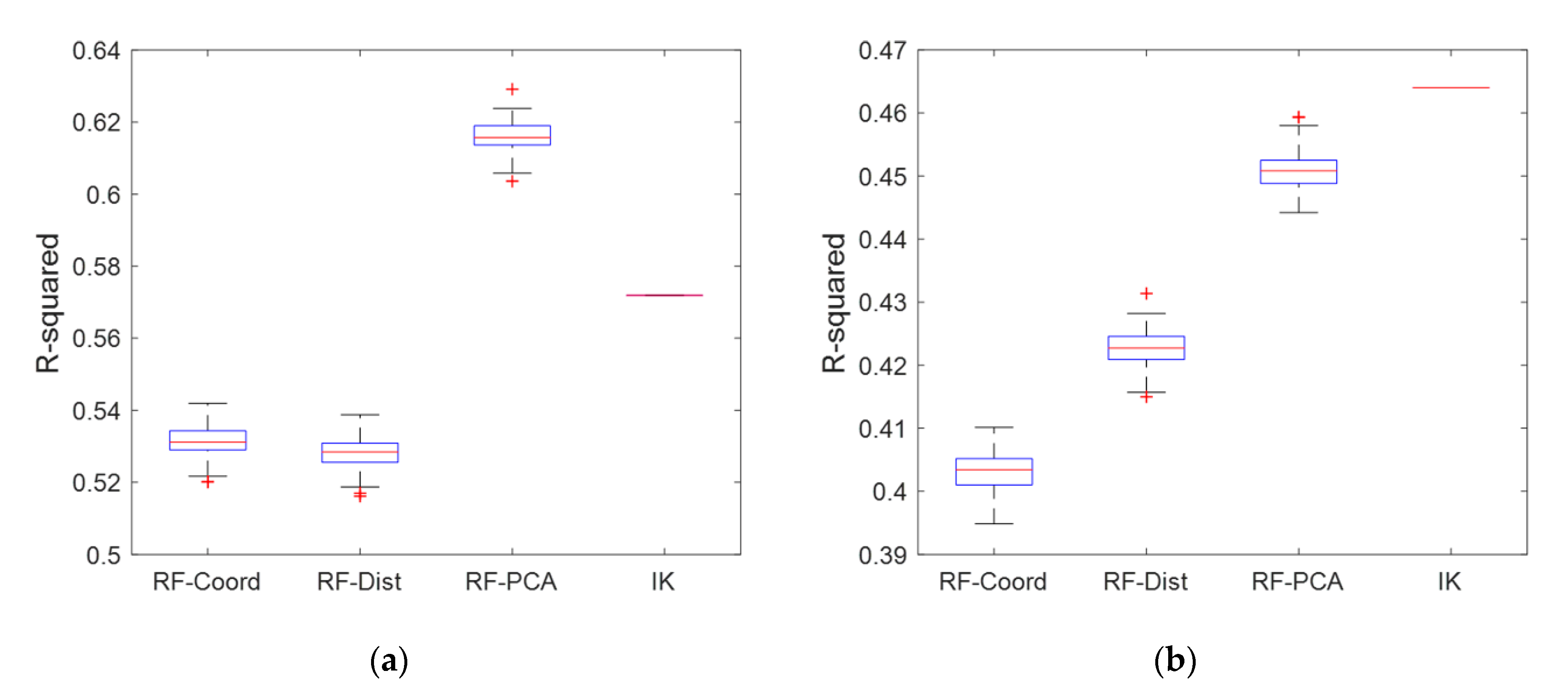Figure 9. Comparison of predictions based on each methodology for the Meuse dataset: predicted zinc concentrations ((a) random forest with coordinate input (RF-Coord); (b) RF with distance input (RF-Dist); (c) RF with principal component analysis (RF-PCA); (d) indicator kriging (IK)) and standard deviation of prediction error ((e) RF-Coord; (f) RF-Dist; (g) RF-PCA; (h) IK).
Figure 9. Comparison of predictions based on each methodology for the Meuse dataset: predicted zinc concentrations ((a) random forest with coordinate input (RF-Coord); (b) RF with distance input (RF-Dist); (c) RF with principal component analysis (RF-PCA); (d) indicator kriging (IK)) and standard deviation of prediction error ((e) RF-Coord; (f) RF-Dist; (g) RF-PCA; (h) IK).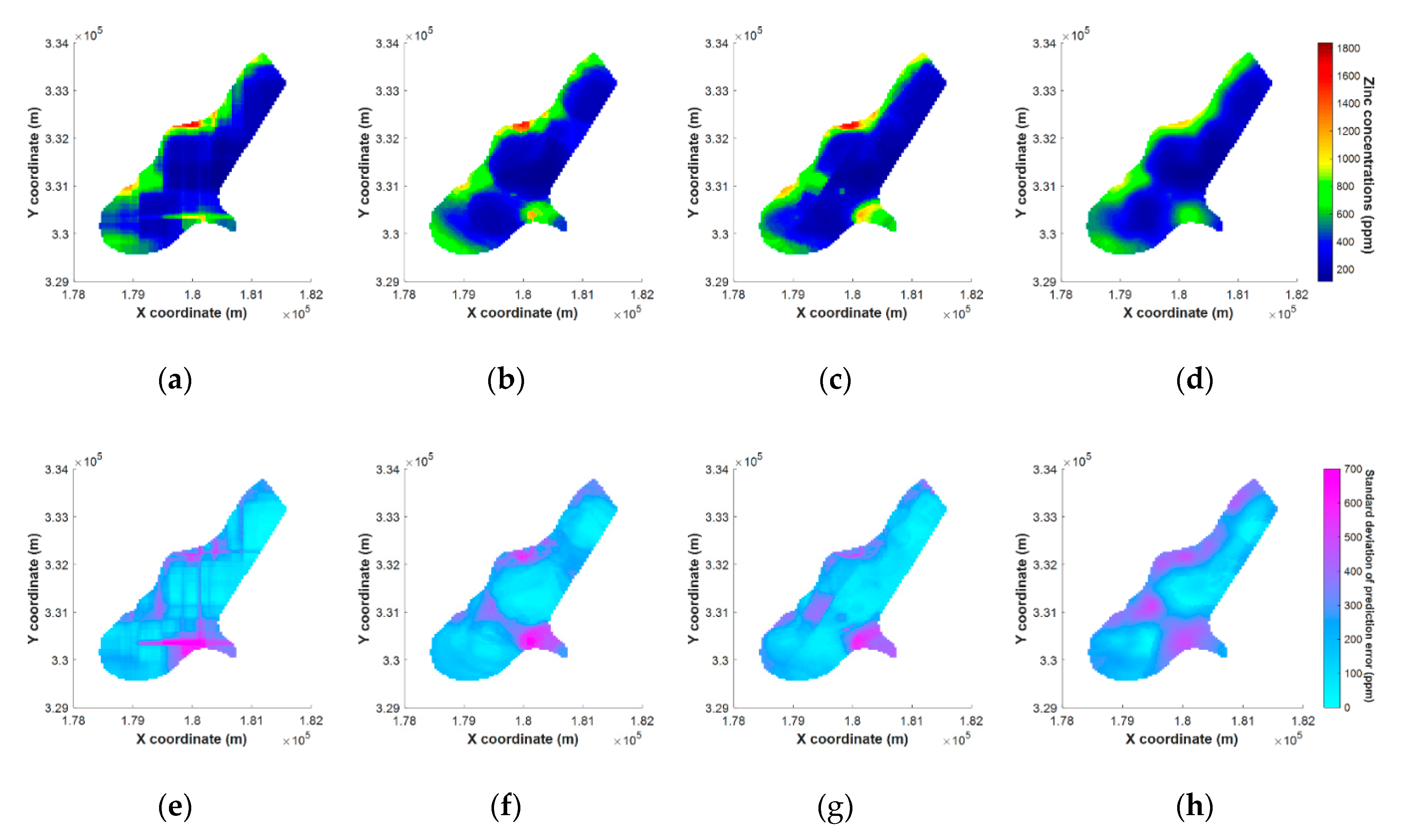Figure 10. Comparison of predictions based on each methodology for Seoul dataset: predicted deposit soil thickness ((a) RF-Coord; (b) RF-Dist; (c) RF-PCA; (d) IK) and standard deviation of prediction error ((e) RF-Coord; (f) RF-Dist; (g) RF-PCA; (h) IK).
Figure 10. Comparison of predictions based on each methodology for Seoul dataset: predicted deposit soil thickness ((a) RF-Coord; (b) RF-Dist; (c) RF-PCA; (d) IK) and standard deviation of prediction error ((e) RF-Coord; (f) RF-Dist; (g) RF-PCA; (h) IK).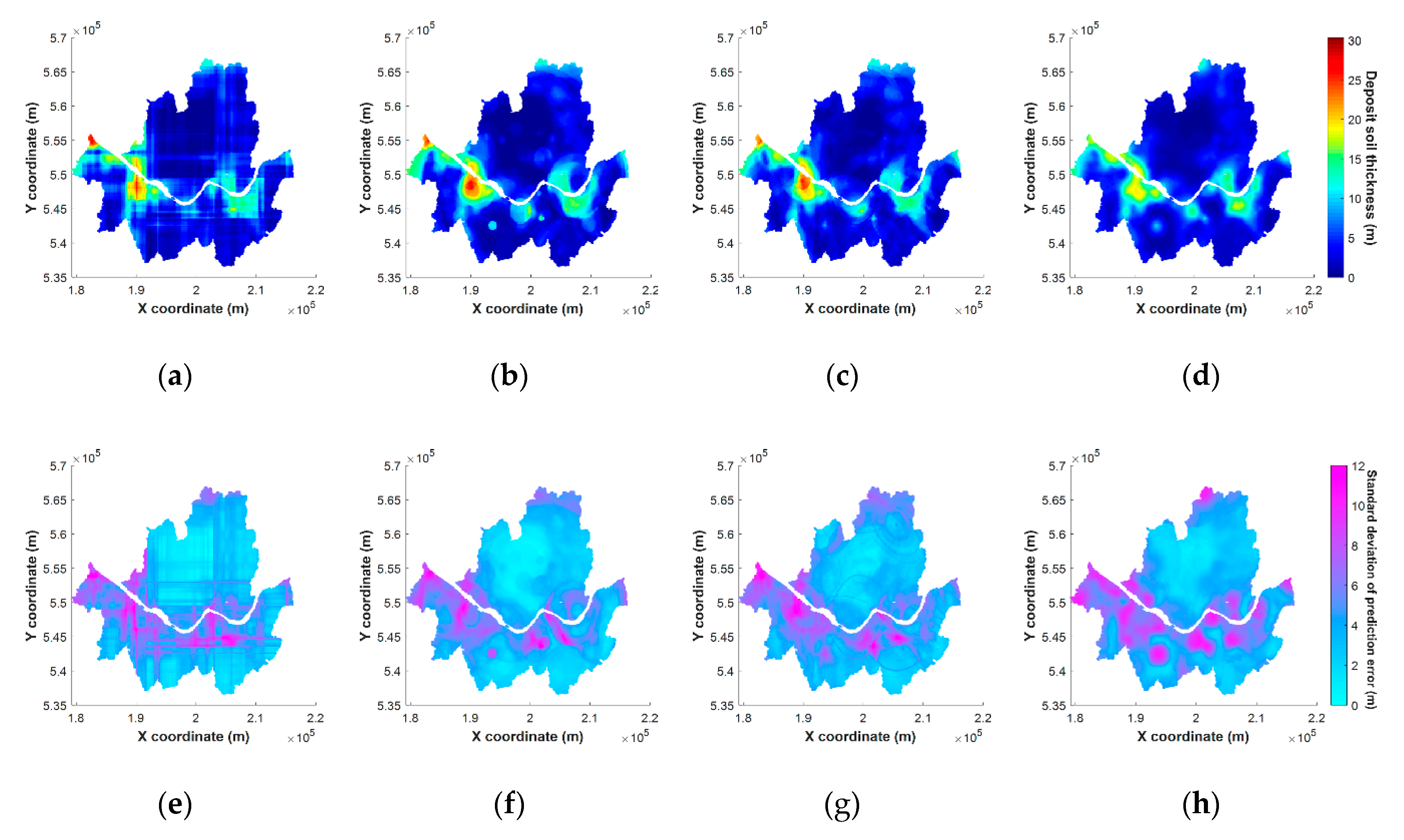Figure 11. Spatial prediction from the RF-PCA using fifteen PCs for the Meuse dataset: (a) Including the third PC and (b) excluding the third PC.
Figure 11. Spatial prediction from the RF-PCA using fifteen PCs for the Meuse dataset: (a) Including the third PC and (b) excluding the third PC.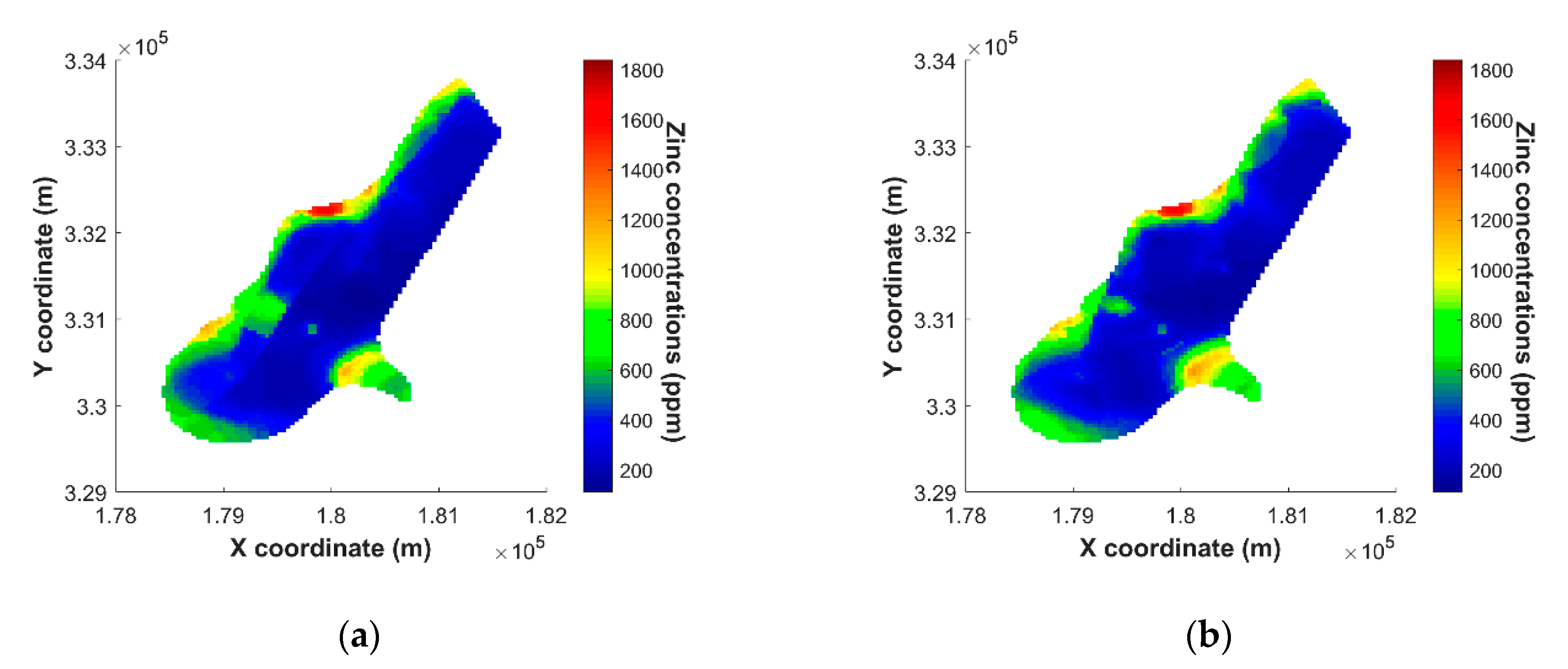Figure 12. Results of five-fold cross-validation of RF-PCA according to the number of PCs increasing for the (a) Meuse dataset and (b) Seoul dataset.
Figure 12. Results of five-fold cross-validation of RF-PCA according to the number of PCs increasing for the (a) Meuse dataset and (b) Seoul dataset.Table 1. List of the method used in the experiment.
Table 1. List of the method used in the experiment.
AlgorithmsInput TypeApplying PCAAbbreviation
Indicator KrigingCoordinateNoIK
Random ForestCoordinateNoRF-Coord
Random ForestDistanceNoRF-Dist
Random ForestDistanceYesRF-PCA
Table 2. Parameters of theoretical variogram models for each dataset.
Table 2. Parameters of theoretical variogram models for each dataset.
DatasetThreshold ValueSemi-Variogram ModelNugget (m²)Sill (m²)Range (m)
Meuse1 (152 ppm)Spherical0.14851.44991392.74
2 (187 ppm)Spherical0.25030.9350832.44
3 (207 ppm)Spherical0.45200.6623817.70
4 (246 ppm)Spherical0.25860.8617778.85
5 (326 ppm)Spherical0.30380.7826728.13
6 (442 ppm)Spherical0.36240.7249765.54
7 (593 ppm)Spherical0.54800.5493882.68
8 (741 ppm)Spherical0.41640.7119826.08
9 (1022 ppm)Exponential0.67120.41461044.20
Seoul1~4 (0 m)Spherical (1st)0.75660.15605282.20
Spherical (2nd) 0.117212172.94
5 (2.6 m)Spherical (1st)0.64590.23654214.28
Exponential (2nd) 0.169213754.17
6 (4.8 m)Exponential (1st)0.33430.26033685.97
Exponential (2nd) 0.41273706.61
7 (7.3 m)Spherical (1st)0.40800.52803343.64
Exponential (2nd) 0.120119061.42
8 (11.1 m)Spherical (1st)0.34100.59193107.19
Exponential (2nd) 0.154919990.08
9 (15.6 m)Spherical0.23640.74432580.06
Table 3. Summary of the results for prediction performance of each methodology with basic statistics.
Table 3. Summary of the results for prediction performance of each methodology with basic statistics.
DatasetParametersRF-CoordRF-DistRF-PCAIKTrue
MeuseMean457.9457.5452.8459.7469.7
Max1510.01416.51425.3958.41839.0
Median427.2396.2383.7435.7326.0
Min145.1132.0144.7133.0113.0
Std.250.1256.2251.2227.8367.1
RMSE251.4251.8230.4247.8-
R-squared0.530.530.620.57-
SeoulMean5.315.035.255.215.34
Max21.0221.2621.2019.3530.34
Median4.103.683.824.032.60
Min0.000.020.130.000.00
Std.4.384.464.283.926.89
RMSE5.165.094.964.93-
R-squared0.400.420.450.46-
Table 4. Summary of the prediction performances of RF-PCA according to the number of the PCs with basic statistics.
Table 4. Summary of the prediction performances of RF-PCA according to the number of the PCs with basic statistics.
DatasetParametersRF-PCA 15RF-PCA 30RF-PCA 50RF-PCA 100True
MeuseMean452.8445.5438.2426.3469.7
Max1425.31227.21123.91010.71839.0
Median383.7386.8388.8373.6326.0
Min144.7147.7158.9168.8113.0
Std.251.2235.6223.7208.2367.1
RMSE230.4243.5246.7257.4-
R-squared0.620.580.570.55-
SeoulMean5.255.024.814.225.34
Max21.2019.8719.7818.2830.34
Median3.823.743.723.202.60
Min0.130.070.210.340.00
Std.4.284.043.843.366.89
RMSE4.965.015.065.26-
R-squared0.450.440.440.43-